Science, Maths & Technology

### Become an OU studentGeometry

Start this free course now. Just create an account and sign in. Enrol and complete the course for a free statement of participation or digital badge if available.

# Try some yourself

## Question 1

Find the area of each of these shapes.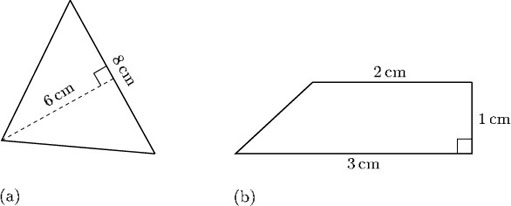(a)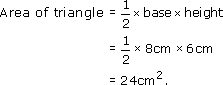(b) The trapezium can be split into a triangle and a rectangle: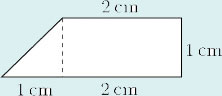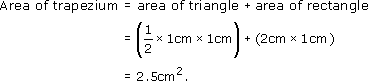## Question 2

A girl is decorating a box by glueing wrapping paper on each face. She wants to put paper on the sides, the top and the bottom, and intends to cut out six pieces of paper and stick them on. Assuming no wastage, calculate what area of paper she will need.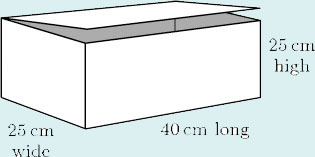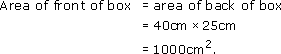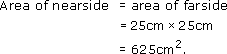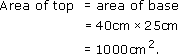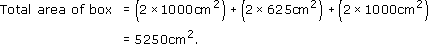Therefore, the amount of material needed is 5250 cm2.

## Question 3

A rug measures 3 m by 2 m. It is to be laid on a wooden floor that is 5 m long and 4 m wide. The floorboards not covered by the rug are to be varnished.

• (a) What area of floor will need to be varnished?

• (b) A tin of varnish covers 2.5 m2. How many tins will be required?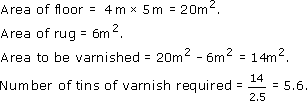So six tins will have to be purchased.

## Question 4

This diagram represents the end wall of a bungalow; the wall contains two windows. The wall is to be treated with a special protective paint. In order to decide how much paint is required, the owner wants to know the area of the wall. Divide the wall up into simple shapes and then find the total area.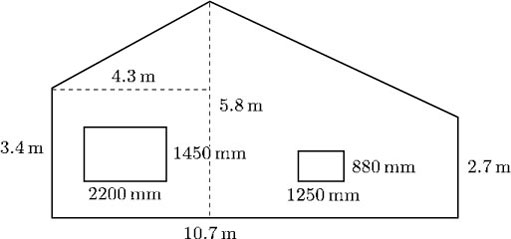The end wall of the bungalow, minus the windows, can be divided into simple shapes as shown.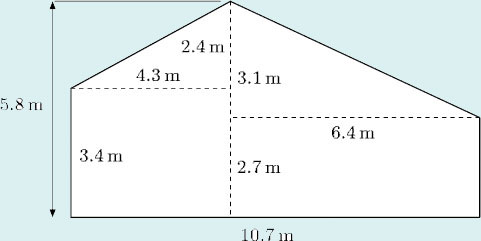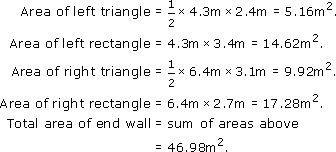The dimensions of the windows, in metres, are 2.2 m by 1.45 m and 1.25 m by 0.88 m, respectively.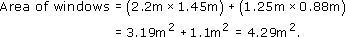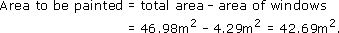## Question 5

The diagram below shows the dimensions of a frame tent. Calculate the amount of canvas needed to make the tent, ignoring the door which is made of different material.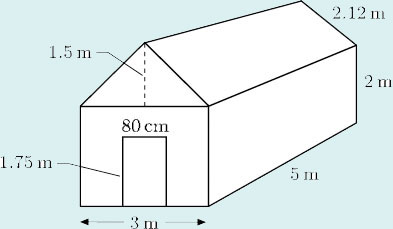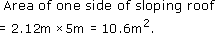Area of one side of tent = 2 m × 5 m = 10 m2.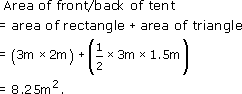Area of door = 0.8 m × 1.75 m = 1.4 m2.

Total area of canvas

• = ( 2 × area of one side of sloping roof )

• + ( 2 × area of side of tent )

• + ( 2 × area of front/back of tent )

• − ( area of door )

• = ( 2 × 10.6 m2 ) + ( 2 × 10 m2 )

• + ( 2 × 8.25 m2 ) − 1.4 m2

• = 56.3 m2.

So 56.3 m2 of canvas are needed.

(In practice, the amount needed will depend upon the width of the canvas and on how many joins there are. It is likely that at least 60 m2 will be needed.)